## Example Questions

### Example Question #3 : How To Find The Ratio Of A Fraction

A pie is made up ofcrust,apples, andsugar, and the rest is jelly. What is the ratio of crust to jelly?

Possible Answers: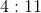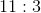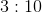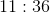Correct answer:Explanation:

A pie is made up ofcrust,apples,sugar, and the rest is jelly. What is the ratio of crust to jelly?

To compute this ratio, you must first ascertain how much of the pie is jelly. This is: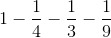Begin by using the common denominator: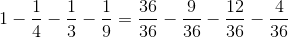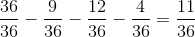So, the ratio of crust to jelly is: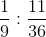This can be written as the fraction: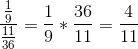, or### Example Question #4 : How To Find The Ratio Of A Fraction

In a solution,of the fluid is water,is wine, and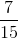is lemon juice. What is the ratio of lemon juice to water?

Possible Answers: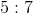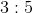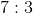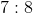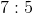Correct answer:Explanation:

This problem is really an easy fraction division. You should first divide the lemon juice amount by the water amount: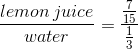Remember, to divide fractions, you multiply by the reciprocal: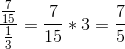This is the same as saying:### Example Question #5 : How To Find The Ratio Of A Fraction

If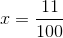and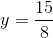, what is the ratio ofto?

Possible Answers: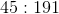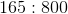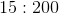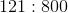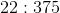Correct answer:Explanation:

To find a ratio like this, you simply need to make the fraction that represents the division of the two values by each other. Therefore, we have: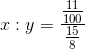Recall that division of fractions requires you to multiply by the reciprocal: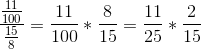which is the same as: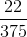This is the same as the ratio:### All ACT Math Resources